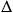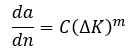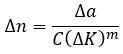# Damage ToleranceMarch 4, 2016 By: Michael Bak

Some time ago, I was called into a meeting to discuss an urgent matter related to failure of a wind turbine.  Two wind turbines were built and started at the same time, and after a period of performance, one of the turbines failed.  The main questions that were asked at the meeting were if the wind turbine that didn’t fail could be started back up, and if so, for how long?

The answer to those questions was found using the damage tolerance approach. Damage tolerance is a marriage of physical inspection and analysis techniques. Inspection is used to determine existing crack size and locations that are input into the simulation. Linear elastic fracture mechanics is then used to determine the stress intensity factor (K) at the crack tip for a given loading. Finally, fatigue analysis describes the change in crack length per cycle (da/dN) as a function of cyclic stress intensity factor (K).

Non-destructive evaluation (NDE) techniques are used to measure crack size without causing additional damage to the structure. Common techniques include ultrasonic, liquid penetrant, radiographic, remote visual inspection, and eddy-current testing.  The flaw size that can be found is dependent on the technique used. NDE is used to set the initial crack length for the damage tolerance calculations. The initial crack should be set to the biggest crack that can be missed by inspection. A commonly assumed initial crack length is 1/32”, which is considered the smallest crack discernable by eye.

Fatigue crack propagation is the combination of linear elastic fracture mechanics (LEFM) and fatigue. In LEFM, the stress at the crack tip is singular based on elasticity theory, which means that the stress is unbounded and tends to infinity. However, a finite stress intensity factor (K) can be derived that describes the strength of the singularity for a given crack size and loading, and this stress intensity factor is used to characterize the near-crack response.

The fatigue part of the damage tolerance approach is typically based on the Paris Law. The Paris Law is a linear fit on a log-log plot to fatigue test data in the form of crack length as a function of cycles (da/dn) versus the change in the stress intensity factor over a cycle (K). Most engineering metals exhibit this linear behavior for crack growth, represented by the linear region (region II) of the plot in Figure 1.

Figure 1: Fatigue Crack Growth Data

## damage1.pngThe Paris Law expression is shown below, with material constants (C, m) defined for a given material.The Paris Law can be re-written to solve numerically for the number of cycles (n) to grow a crack an additional length (a) subjected to a given cyclic loading (K) for a given material (C, m).Therefore, the general procedure for performing a damage tolerance fatigue crack propagation calculation is to find theK for a structure with a given crack and given cyclic loading, typically performed using a finite element analysis. The crack is extended a small amount, the simulation is repeated, and the number of cycles found from the Paris Law to extend the crack is calculated. This calculation is repeated, continually extending the crack further, recalculatingK, and determining the number of cycles to propagate the crack, until the structure fails or the crack reaches a critical length.

The main challenges in this type of modeling are determining the direction of crack propagation, and how to efficiently model a propagating crack in which the crack path is not known ahead of time. The crack direction is typically chosen to be perpendicular to the maximum principal stress at the crack tip. Modeling the changing crack path using finite elements is the more difficult challenge.

A number of modeling approaches are available for use in crack propagation analyses. The most basic and user-intensive is to manually re-mesh the structure to extend the crack. Another approach is to use a mesh-morphing procedure to move the nodes and separate the elements to include the growing crack. A third approach is to model the crack separately from the finite element mesh.

The eXtended Finite Element Method (XFEM) approach falls into the third category. XFEM works by enriching the degrees of freedom and applying additional displacement functions in elements where a crack passes through. The additional displacement functions account for the jump in displacements across a crack discontinuity. The crack can grow independently of the original mesh, and thus eliminates the necessity of re-meshing crack tip regions. And, using a singularity-based XFEM, stress intensity factors can be automatically calculated, and thus fatigue crack growth simulations can be automated.

An example is shown in the two-dimensional analysis using ANSYS Mechanical APDL in Figure 2. An initial crack is defined as shown. The XFEM-based fatigue crack growth analysis is performed, using a procedure that calculates the number of cycles to extend the crack one element in each substep using a Paris Law material model. Figure 3 shows how the crack turns and eventually propagates downward based on the direction of the first principal stress field. An animation of the crack propagation is shown in Figure 4.

Figure 2: 2D XFEM Analysis with Starting Initial Crack Shown

## damage2.pngFigure 3: Crack Propagating Through the Mesh

## damage3.pngFigure 4: Animation of Crack Propagation

## xfem_test.gifIn addition to predicting the path of the crack, the number of cycles of loading as a function of crack length is automatically generated and shown in Figure 5. The value ofK as a function of crack length is also available and shown in Figure 6. Note thatK can change with crack length due to the changing crack configuration and redistribution of the loading due to the propagating crack.

Figure 5: Cycles Versus Crack Length

## damage5.pngFigure 6: Delta K Versus Crack Length

## damage6.pngResults from a fatigue crack growth calculation can be used to estimate remaining life in the structure. Once cycles to failure is determined, an inspection interval representing a percentage of the predicted cycles to failure can be planned, at which time a new inspection is performed, and the fatigue crack growth calculation updated. Using the damage tolerance approach, the life of structures can be extended. This is the exact procedure that was used to enable the surviving wind turbine described above to be restarted.

Have you ever performed a fatigue crack propagation and used the damage tolerance approach? I welcome your comments below.